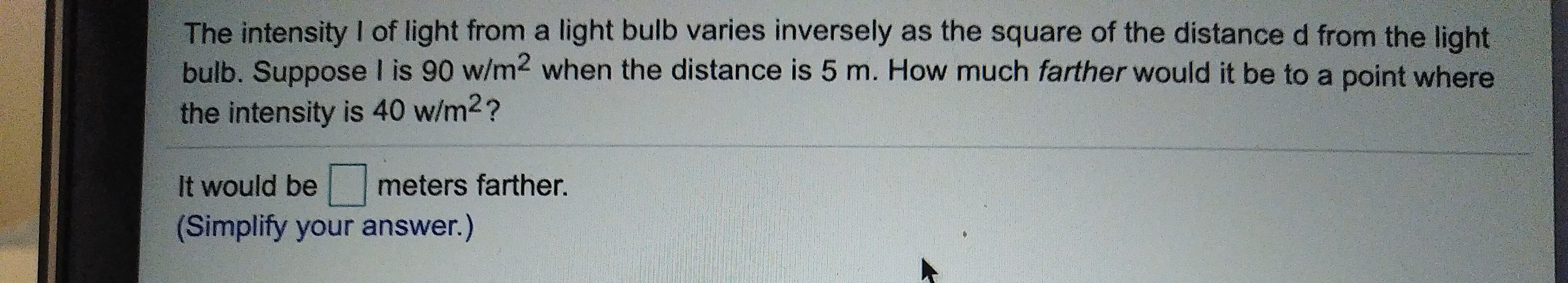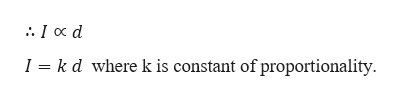The intensity I of light from a light bulb varies inversely as the square of the distanced from the lightbulb. Suppose I is 90 w/m2 when the distance is 5 m. How much farther would it be to a point wherethe intensity is 40 w/m2?meters farther.It would be(Simplify your answer.)

Questionhelp_outlineImage TranscriptioncloseThe intensity I of light from a light bulb varies inversely as the square of the distanced from the light bulb. Suppose I is 90 w/m2 when the distance is 5 m. How much farther would it be to a point where the intensity is 40 w/m2? meters farther. It would be (Simplify your answer.) fullscreen
Step 1

Given,

The intensity I of light from a light bulb varies inversely as the square of the distance d from the light bulb.help_outlineImage Transcriptionclose1 k d where k is constant of proportionality. fullscreen
Step 2

When I = 90w/m2 then d = 5 m.

So, 90 = k (5)

k = 18

Therefore, I = 18d

Again, when I = 40w/m2 then

...

Want to see the full answer?

See Solution

Want to see this answer and more?

Our solutions are written by experts, many with advanced degrees, and available 24/7

See Solution
Tagged in

Other